### Finite-Difference Approximations of Derivatives

The FD= and FDHESSIAN= options specify the use of finite-difference approximations of the derivatives. The FD= option specifies that all derivatives are approximated using function evaluations, and the FDHESSIAN= option specifies that second-order derivatives are approximated using gradient evaluations.

Computing derivatives by finite-difference approximations can be very time-consuming, especially for second-order derivatives based only on values of the objective function (FD= option). If analytical derivatives are difficult to obtain (for example, if a function is computed by an iterative process), you might consider one of the optimization techniques that use first-order derivatives only (QUANEW, DBLDOG, or CONGRA). In the expressions that follow,denotes the parameter vector,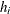denotes the step size for the ith parameter, and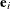is a vector of zeros with a 1 in the ith position.

#### Forward-Difference Approximations

The forward-difference derivative approximations consume less computer time, but they are usually not as precise as approximations that use central-difference formulas.

• For first-order derivatives, n additional function calls are required: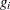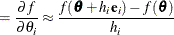• For second-order derivatives based on function calls only (Dennis and Schnabel, 1983, p. 80),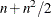additional function calls are required for dense Hessian: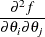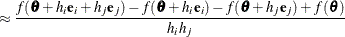• For second-order derivatives based on gradient calls (Dennis and Schnabel, 1983, p. 103), n additional gradient calls are required: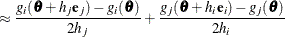#### Central-Difference Approximations

Central-difference approximations are usually more precise, but they consume more computer time than approximations that use forward-difference derivative formulas.

• For first-order derivatives, 2n additional function calls are required: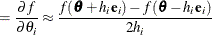• For second-order derivatives based on function calls only (Abramowitz and Stegun, 1972, p. 884),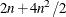additional function calls are required.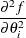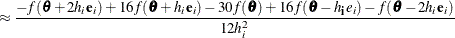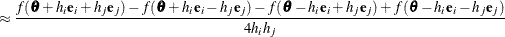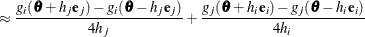You can use the FDIGITS= option to specify the number of accurate digits in the evaluation of the objective function. This specification is helpful in determining an appropriate interval size h to be used in the finite-difference formulas.

The step sizes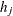,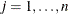are defined as follows:

• For the forward-difference approximation of first-order derivatives that use function calls and second-order derivatives that use gradient calls,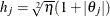.

• For the forward-difference approximation of second-order derivatives that use only function calls and all central-difference formulas,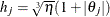.

The value of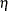is defined by the FDIGITS= option:

• If you specify the number of accurate digits by using FDIGITS=r,is set to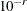.

• If you do not specify the FDIGITS= option,is set to the machine precision.